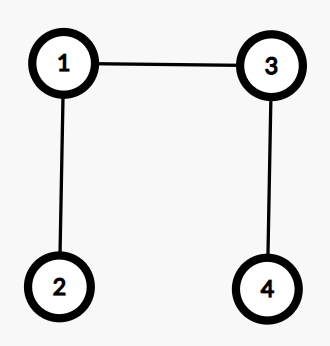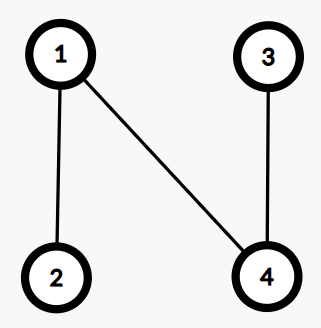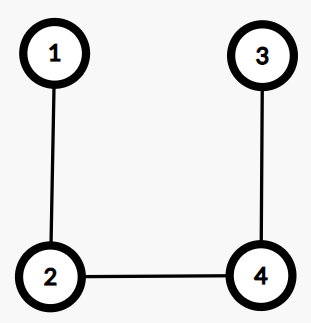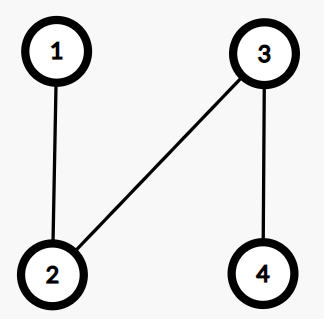2021-01-16 23:32:52

1. 将$1\sim n$分为$k$个区间，每段区间的长度不小于$2$。
2. 每段区间内部只有一种连边的方法，接着我们用$k-1$条边将这$k$个连通块连接起来。注意不能再有类似$(i,i+1)$这样的连边，我们称包含这种连边的情况为不合法情况。\begin{aligned} \prod_{j=1}^ia_j\times (\sum_{k=1}^ia_k)^{i-2}=\prod a_i\times n^{i-2} \end{aligned}

$$A(x)+A(x)^2+A(x)^3+\cdots=\frac{1}{1-A(x)}-1$$

\begin{aligned} \frac{1}{1-A(x)}-1&=x^2+x^3+x^4+\cdots\\ &=\frac{1}{1-x}-1-x \end{aligned}

$$A(x)=\frac{x^2}{x^2-x+1}$$

\begin{aligned} B(x)&=\sum_{j\geq 2}nx^jA_j\\ dp[n][i]&=B(x)^i[x^n]\\ ans&=\sum_i \frac{dp[n][i]}{n^2}\\ &=\sum_i\frac{B(x)^i[x^n]}{n^2}\\ &=\frac{\frac{1}{1-B(x)}[x^n]}{n^2} \end{aligned}

• star
首页